Test: Complex Number- 1

# Test: Complex Number- 1

Test Description

## 5 Questions MCQ Test Mathematics for Airmen Group X | Test: Complex Number- 1

Test: Complex Number- 1 for Airforce X Y / Indian Navy SSR 2023 is part of Mathematics for Airmen Group X preparation. The Test: Complex Number- 1 questions and answers have been prepared according to the Airforce X Y / Indian Navy SSR exam syllabus.The Test: Complex Number- 1 MCQs are made for Airforce X Y / Indian Navy SSR 2023 Exam. Find important definitions, questions, notes, meanings, examples, exercises, MCQs and online tests for Test: Complex Number- 1 below.
Solutions of Test: Complex Number- 1 questions in English are available as part of our Mathematics for Airmen Group X for Airforce X Y / Indian Navy SSR & Test: Complex Number- 1 solutions in Hindi for Mathematics for Airmen Group X course. Download more important topics, notes, lectures and mock test series for Airforce X Y / Indian Navy SSR Exam by signing up for free. Attempt Test: Complex Number- 1 | 5 questions in 10 minutes | Mock test for Airforce X Y / Indian Navy SSR preparation | Free important questions MCQ to study Mathematics for Airmen Group X for Airforce X Y / Indian Navy SSR Exam | Download free PDF with solutions
 1 Crore+ students have signed up on EduRev. Have you?
Test: Complex Number- 1 - Question 1

### If a,b (a≠b), are the real roots of the equation (k + 1)(x2 + x + 1)2 + (k - 1)(x4 + x2 + 1) = 0, k ≠ 1, 0.  Then the product of the roots is

Detailed Solution for Test: Complex Number- 1 - Question 1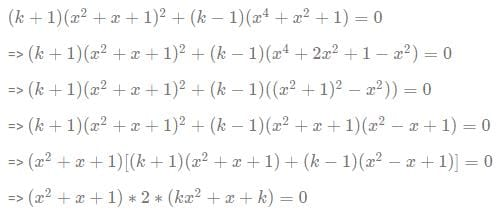Since the equation (x+  x + 1) = 0 . oes not have any real roots, the roots of the original equation will be the root of the equation (kx2 + x + k) = 0

Hence product of the roots = k/k = 1

Test: Complex Number- 1 - Question 2

### Polar form of a complex number is

Test: Complex Number- 1 - Question 3

### |z1 + z2 | =

Detailed Solution for Test: Complex Number- 1 - Question 3

|z1 + z2|=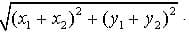.
|z1| + |z2|=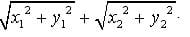.

We have to prove that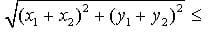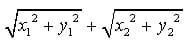is true.
Square both sides.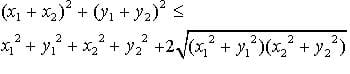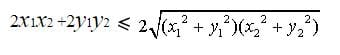Square both sides again.
2x1x2y1y2 ≦ x12y2y12x22 and we get
0 ≦ (y1x2 - x1y2)2.
It is true because x1, x2y1y2 are all real.

Test: Complex Number- 1 - Question 4

|z1 - z2 | =

Test: Complex Number- 1 - Question 5

a2 + b2

Detailed Solution for Test: Complex Number- 1 - Question 5

(a + ib) x (a - ib)

= a2 - i2b2

we know i2=-1

so, equation becomes a2 -(-1)b2 =

a2 + b2

## Mathematics for Airmen Group X

131 videos|195 docs|197 tests
 Use Code STAYHOME200 and get INR 200 additional OFF Use Coupon Code
Information about Test: Complex Number- 1 Page
In this test you can find the Exam questions for Test: Complex Number- 1 solved & explained in the simplest way possible. Besides giving Questions and answers for Test: Complex Number- 1, EduRev gives you an ample number of Online tests for practice

## Mathematics for Airmen Group X

131 videos|195 docs|197 tests